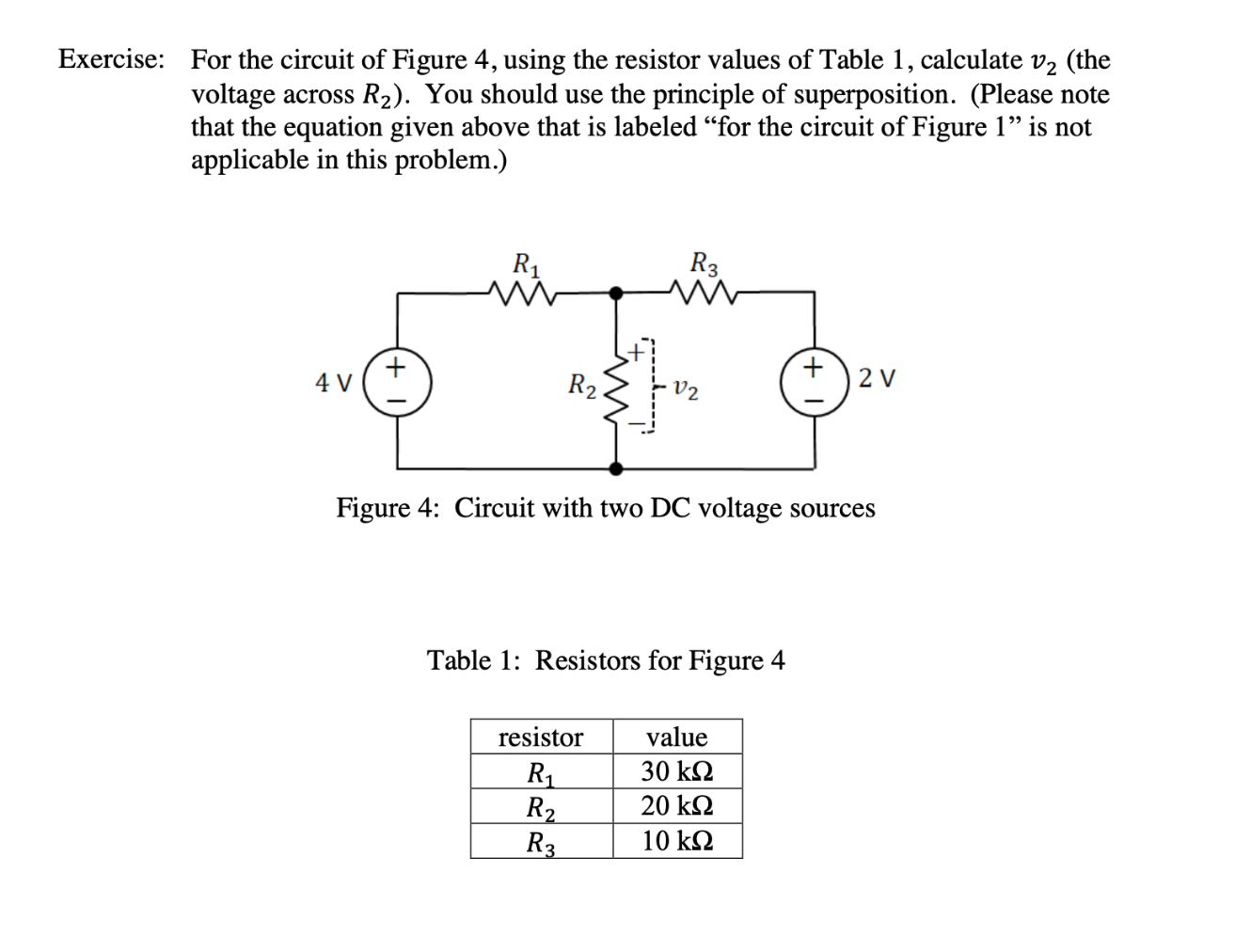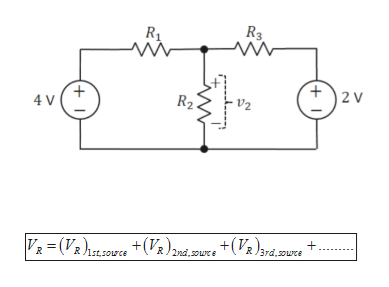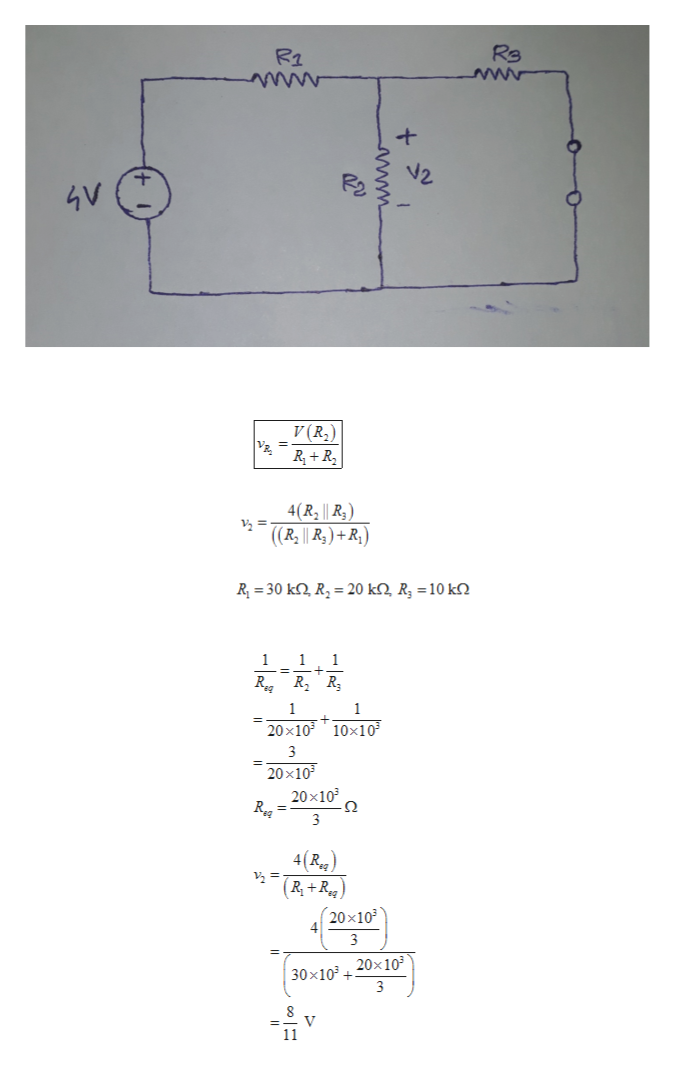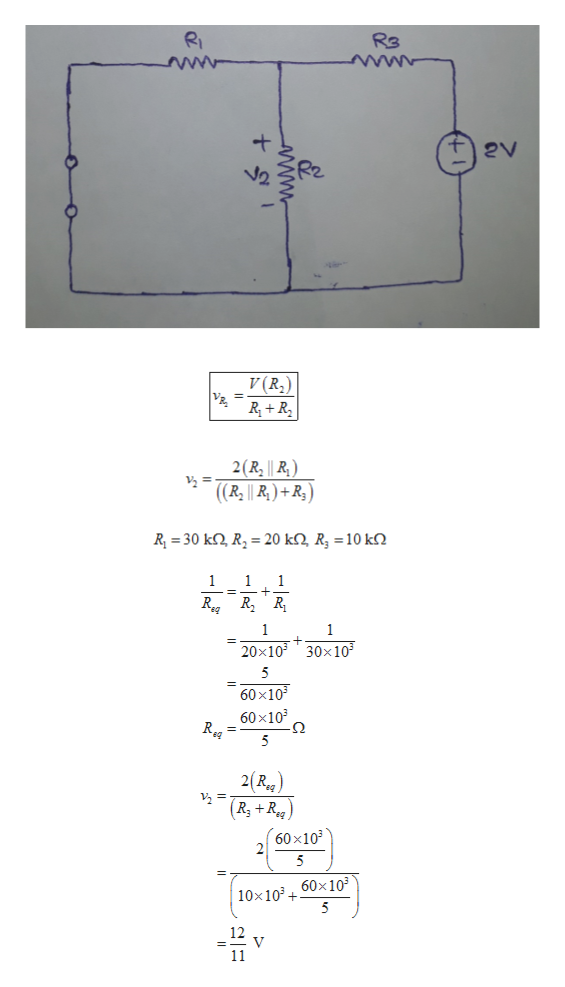Exercise:For the circuit of Figure 4, using the resistor values of Table 1, calculate v2 (thevoltage acrossthat the equation given above that is labeled "for the circuit of Figure 1" is notapplicable in this problem.)R2). You should use the principle of superposition. (Please noteR3R1W2 V4 VR2Figure 4: Circuit with two DC voltage sourcesTable 1: Resistors for Figure 4resistorvalue30 k20 k2R2R310 kww

Questionhelp_outlineImage TranscriptioncloseExercise: For the circuit of Figure 4, using the resistor values of Table 1, calculate v2 (the voltage across that the equation given above that is labeled "for the circuit of Figure 1" is not applicable in this problem.) R2). You should use the principle of superposition. (Please note R3 R1 W 2 V 4 V R2 Figure 4: Circuit with two DC voltage sources Table 1: Resistors for Figure 4 resistor value 30 k 20 k2 R2 R3 10 k ww fullscreen
Step 1

The superposition theorem for any linear circuit states that the total current or voltage in any part of the circuit equals the algebraic sum of currents or voltages produced by each independent source separately.

The mathematical form of superposition theorem:help_outlineImage TranscriptioncloseR3 2 V R2 -V2 4 V V(V.oU V)2ni.ore +V%)syd.ure 3rd,ouce R lst,sorce + 1 fullscreen
Step 2

Consider only 4 V independent source acts on the circuit.

Therefore, the circuit becomes

Now, the voltage across R2 can be calculated using voltage division rule.

The mathematical formula for voltage division rule is

Where vR2 is the voltage across R2 resistance, V is the independent source voltage.

Therefore,

The given value of R1, R2, R3 is

The equivalent resistance of R2 and R3 is

This is the voltage across R2 when only 4 V source is present.help_outlineImage TranscriptioncloseRe R2 4V V(R R R 4(R2 || R, ) 2 = ((R|| R)+R R 30 k R2 20 kQ, R, = 10 k 1 1 1 1 1 20x103 10x103 3 20x10 20x103 о 3 R 4(R.g (R+R (20x10 4 3 30x10320x103 3 V 11 www fullscreen
Step 3

Consider only 2 V independent source acts on the circuit.

Therefore, the circuit becomes

Now, the voltage across R2 can be calculated using voltage division rule.

The mathematical formula for voltage division rule is

Where vR2 is the voltage across R2 resistance, V is the independent source voltage.

Therefore,

The given value o...help_outlineImage TranscriptioncloseRI RB www 2V v (R2) RR 2(R, || R) 2 = ((R || R)+R) R 30 k R 20 k2, R, = 10 kQ 1 1 1 RegRR 1 1 30x10 20x10 5 60 x103 60x103 = 2(Rg (60x10 10x10360x10 5 12 V 11 www fullscreen

Want to see the full answer?

See Solution

Want to see this answer and more?

Our solutions are written by experts, many with advanced degrees, and available 24/7

See Solution
Tagged in

Electrical Engineering